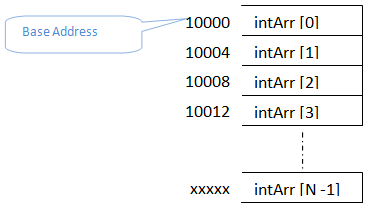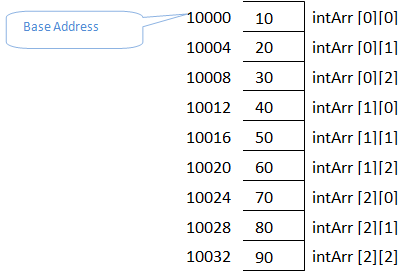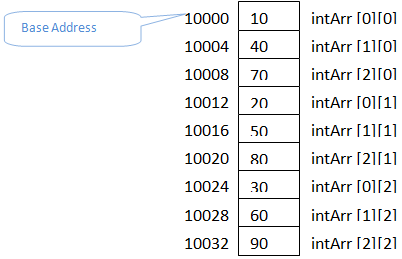# Array Memory Allocation in C Programming

We have already discussed that whenever an array is declared in the program, contiguous memory to it elements are allocated. Initial address of the array – address of the first element of the array is called base address of the array. Each element will occupy the memory space required to accommodate the values for its type, i.e.; depending on elements datatype, 1, 4 or 8 bytes of memory is allocated for each elements. Next successive memory address is allocated to the next element in the array. This process of allocating memory goes on till the number of element in the array gets over.

## One Dimensional Array

Below diagram shows how memory is allocated to an integer array of N elements. Its base address – address of its first element is 10000. Since it is an integer array, each of its element will occupy 4 bytes of space. Hence first element occupies memory from 10000 to 10003. Second element of the array occupies immediate next memory address in the memory, i.e.; 10004 which requires another 4 bytes of space. Hence it occupies from 10004 to 10007. In this way all the N elements of the array occupies the memory space.If the array is a character array, then its elements will occupy 1 byte of memory each. If it is a float array then its elements will occupy 8 bytes of memory each. But this is not the total size or memory allocated for the array. They are the sizes of individual elements in the array. If we need to know the total size of the array, then we need to multiply the number of elements with the size of individual element.

i.e.; Total memory allocated to an Array = Number of elements * size of one element

Total memory allocated to an Integer Array of N elements = Number of elements * size of one element
= N * 4 bytes
= 10 * 4 bytes = 40 Bytes, where N = 10
= 500 * 4 bytes = 2000 Bytes, where N = 500

Total memory allocated to an character Array of N elements= Number of elements * size of one element
= N * 1 Byte
= 10 * 1 Byte = 10 Bytes, where N = 10
= 500 * 1 Byte = 500 Bytes, where N=500
This is how memory is allocated for the single dimensional array.

## Multidimensional Array

In the case of multidimensional array, we have elements in the form of rows and columns. Here also memories allocated to the array are contiguous. But the elements assigned to the memory location depend on the two different methods:

## Row Major Order

Let us consider a two dimensional array to explain how row major order way of storing elements works. In the case of 2D array, its elements are considered as rows and columns of a table. When we represent an array as intArr[i][j], the first index of it represents the row elements and the next index represents the column elements of each row. When we store the array elements in row major order, first we will store the elements of first row followed by second row and so on.  Hence in the memory we can find the elements of first row followed by second row and so on. In memory there will not be any separation between the rows. We have to code in such a way that we have to count the number of elements in each row depending on its column index. But in memory all the rows and their columns will be contiguous. Below diagram will illustrate the same for a 2D array of size 3X3 i.e.; 3 rows and 3 columns.

Array indexes always start from 0. Hence the first element of the 2D array is at intArr. This is the first row-first column element. Since it is an integer array, it occupies 4 bytes of space. Next memory space is occupied by the second element of the first row, i.e.; intArr  – first row-second column element. This continues till all the first row elements are occupied in the memory. Next it picks the second row elements and is placed in the same way as first row. This goes on till all the elements of the array are occupies the memory like below. This is how it is placed in the memory. But seeing the memory address or the value stored in the memory we cannot predict which is the first row or second row or so.Total size/ memory occupied by 2D array is calculated as

Total memory allocated to 2D Array = Number of elements * size of one element
= Number of Rows * Number of Columns * Size of one element

Total memory allocated to an Integer Array of size MXN = Number of elements * size of one element
=M Rows* N Columns * 4 Bytes
= 10*10 * 4 bytes = 400 Bytes, where M =N = 10
= 500*5 *4 bytes= 10000 Bytes, where M=500 and N= 5

Total memory allocated to an character Array of N elements= Number of elements * size of one element
= M Rows* N Columns * 1 Byte
= 10*10 * 1 Byte = 100 Bytes, where N = 10
= 500*5 * 1 Byte = 2500 Bytes, where M=500 and N= 5

## Column Major Order

This is the opposite method of row major order of storing the elements in the memory. In this method all the first column elements are stored first, followed by second column elements and so on.Total size/ memory occupied by 2D array is calculated as in the same way as above.

Total memory allocated to 2D Array = Number of elements * size of one element
= Number of Rows * Number of Columns * Size of one element

Total memory allocated to an Integer Array of size MXN = Number of elements * size of one element
=M Rows* N Columns * 4 Bytes
= 10*10 * 4 bytes = 400 Bytes, where M =N = 10
= 500*5 *4 bytes= 10000 Bytes, where M=500 and N= 5

Total memory allocated to an character Array of N elements= Number of elements * size of one element
= M Rows* N Columns * 1 Byte
= 10*10 * 1 Byte = 100 Bytes, where N = 10
= 500*5 * 1 Byte = 2500 Bytes, where M=500 and N= 5
If an array is 3D or multidimensional array, then the method of allocating memory is either row major or column major order. Whichever is the method, memory allocated for the whole array is contiguous and its elements will occupy them in the order we choose – row major or column major. The total size of the array is the total number of elements * size of one element.

Translate »